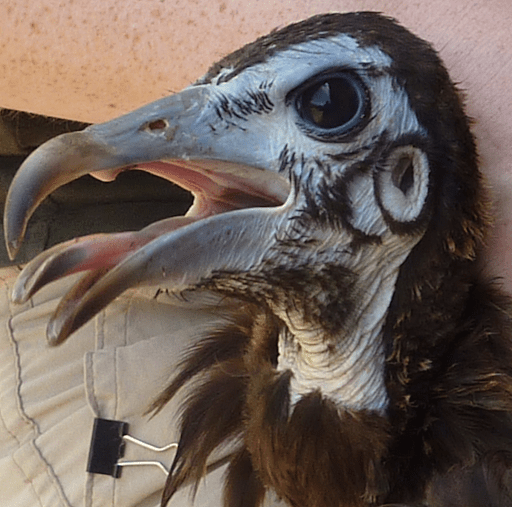# Enigmatic Code

Programming Enigma Puzzles

## Puzzle #13: Snail partySam has four pet snails. She puts one of them at each corner of a square ABCD with sides 2 metres long. Being very friendly, each snail moves towards its neighbour, snail A to snail B, B to C, and so on, at all times pointing directly towards that neighbour. If each snail moves at a constant speed of 2 metres per hour, how long will it be before they meet?

[puzzle#13]

### 5 responses to “Puzzle #13: Snail party”

1.Jim Randell 20 July 2019 at 9:18 am

This is known as a Mice Problem [ link ].

The snails will travel along a logarithmic spiral and meet in the centre of the square, having travelled a distance equal to the side of the square, i.e. 2m. As they travel at 2m/hour this will take 1 hour.

At least that’s what would happen if the snails were points of negligible size. As the snails have non-negligible size (in the diagram in New Scientist the are apparently about half a metre long!), they will collide before reaching the centre, so they will meet in less than 1 hour.

Solution: The snails will meet in just under an hour.

Here is a diagram of the paths, it is generated using the simple plotting library that I use to generate some of the diagrams for the puzzles:Run: [ @repl.it ]

The plotting library is available on GitHub [ link ].

2.Tony Fulford 22 July 2019 at 12:54 pm

It is a good thing that these snails do have a finite size or they would spin faster and faster as they approached one another eventually reaching infinite angular velocity when they meet. The poor things would be infinitely dizzy!

3.Robert Brown 25 July 2019 at 4:44 pm

I saw this puzzle in New Scientist, and solved it by drawing short linear sections. Superimposing your plot over my drawing it fits exactly. Wolfram accepts this method, calling it WHIRL. See here http://mathworld.wolfram.com/Whirl.html

4.Mark Purcell 3 August 2019 at 4:54 pm

Another way to solve is work out the magnitude of the velocity component of a snail towards the centre of the square, which always remains constant as the ‘square’ formed by the snails shrinks and rotates. That magnitude is sqrt(2)m/h. The distance travelled is the original distance to the centre of the square, which is sqrt(2)m. So the time is distance divided by speed, i.e. 1 hour (or as noted very slightly less due to the finite size of the snails).

•Jim Randell 3 August 2019 at 10:31 pm

Or we can consider that at any instant each snail is moving directly towards its quarry, and in that instant the quarry is moving in a perpendicular direction, so that the component of its velocity in the direction of the snail chasing it is zero. So each snail is advancing on its quarry at its speed over the ground, and the quarry is not escaping.

This means that the distance that the chasing snail travels is the same as if the quarry isn’t moving at all, i.e. the length of a side of the square = 2m. The snails travel at 2m/h, so the time taken for the chase is 1h.

This site uses Akismet to reduce spam. Learn how your comment data is processed.